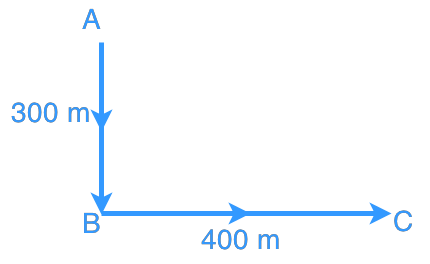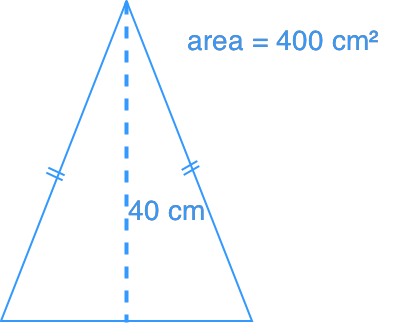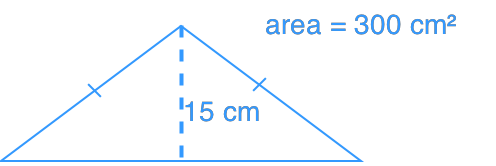# Applications of pythagorean theorem#### All in One Place

Everything you need for better grades in university, high school and elementary.#### Learn with Ease

Made in Canada with help for all provincial curriculums, so you can study in confidence.#### Instant and Unlimited Help

0/2
##### Intros
###### Lessons
1. i) What is Pythagorean Theorem?

ii) How to use Pythagorean Theorem to find sides of a triangle?

2. What are Pythagorean Triples?
0/3
##### Examples
###### Lessons
1. An aeroplane flew from point A to point B, and then to Point C. How far is the plane from point A? Refer to the diagram below.1. A 2.5 m long ladder is put against a wall. If the base of the ladder is 1.25 m away from the wall, what is the length from the ground to the point where the top of ladder touches the wall?
1. Which triangle has a longer perimeter?
Triangle ATriangle B0%
##### Practice
###### Topic Notes
We will need to apply Pythagorean Theorem often in our daily life. In this lesson, we will focus on tackling some Pythagorean Theorem word problems.